# Exploring Geometrical Figures Solution of TS & AP Board Class 8 Mathematics

###### Exercise 8.1

Question 1.

Name five pairs of congruent objects, you use daily.

Congruent Objects have the same size, same shape and same angle. If you place a congruent object in front of a mirror, the image that you see is congruent or "equal" to the object. They completely overlap each other.

Any five pairs of congruent objects you use daily are:

a) Biscuits of same pack

b) All A4 sheets

c) Two bangles

d) Two balls of same brand and same size

e) Two earrings of the same set

Question 2.

Draw two congruent figures. Are they similar? Explain.

We know that two figures are “congruent” if they have the same size, same shape and same angle.

When two figures are “similar”, their angles are same. But that does not mean they have to be congruent. Whereas “Congruent” figures are “always similar”.

Example 1: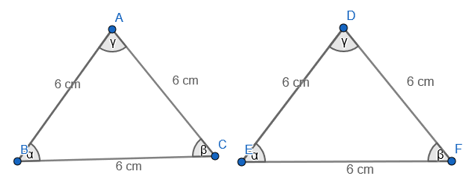Above two figures are congruent as they have same size, same shape and same angle and all congruent figures are similar.

Example 2: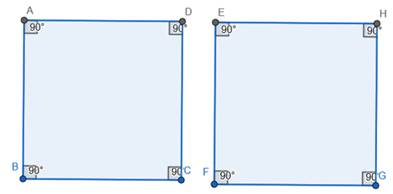Figures given above are similar as they have same angles but are not congruent.

Question 3.

Take two similar shapes. If you slide rotate or flip one of them, does the similarity remain?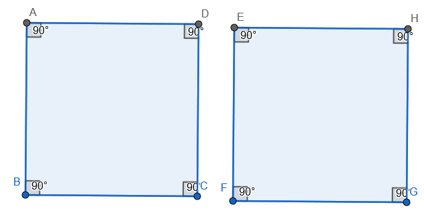Two figures shown above are similar to each other as they have same angles.

On rotating figure on left by 180, we get: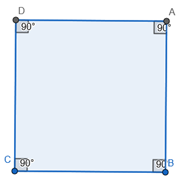We see that the rotated figure is similar to figure on the right.

Question 4.

If Δ ABC ≅ Δ NMO, name the congruent sides and angles.

Given: Δ ABC ≅ Δ NMO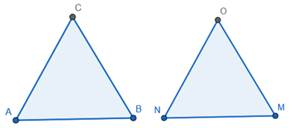Congruent sides:

a) AB = NM

b) BC = MO

c) AC = NO

Congruent angles:

a) ∠A = ∠N

b) ∠B = ∠M

c) ∠C = ∠O

Question 5.

State whether the following statements are true. Explain with reason.

Two squares of side 3 cm each and one of them rotated through 45o are congruent.

True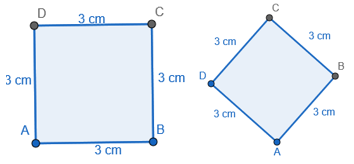On rotating the square by 45, the length of side, angle and shape remains the same. Hence, they are congruent.

Question 6.

State whether the following statements are true. Explain with reason.

Any two right triangles with hypotenuse 5cm, are congruent.

True

For a right angled triangle to have hypotenuse equal to 5 cm, the other two sides have to be 3 cm and 4 cm as the only possible way. As,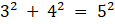by Pythagoras theorem for Right angled triangles. Thus, any two right triangles with hypotenuse 5cm have same angles and are congruent.

Question 7.

State whether the following statements are true. Explain with reason.

Any two circles of radii 4 cm each are congruent.

True

Any two circles of radii 4 cm would completely overlap each other when put front of mirror due to same radii of 4 cm. Hence they are congruent.

Question 8.

State whether the following statements are true. Explain with reason.

Two equilateral triangles of side 4 cm each but labeled as ΔABC and ΔLHN are not congruent.

False

Two equilateral triangles of side 4 cm and angle 60each but labeled as ΔABC and ΔLHN would still be congruent as they have same sides and same angles.

Question 9.

State whether the following statements are true. Explain with reason.

Mirror image of a polygon is congruent to the original.

True

Mirror image of a polygon would have same sides and angles as the original and thus, is congruent to the original.

Question 10.

Draw a polygon on a square dot sheet. Also draw congruent figures in different directions and mirror image of it.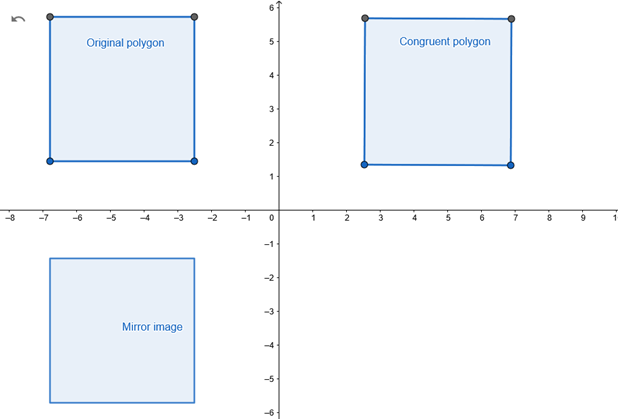Question 11.

Using a square dot sheet or a graph sheet draw a rectangle and construct a similar figure.

Find the perimeter and areas of both and compare their ratios with the ratio of their corresponding sides.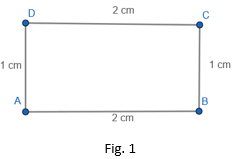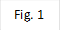Perimeter = 2(l + b)

= 2(2 + 1)

= 2(3)

= 6 cm

Area = l×b

= 2×1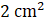A figure similar to above figure is shown below: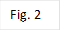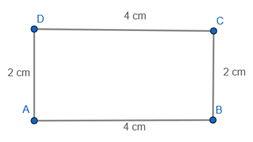Perimeter =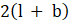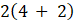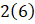= 12 cm

Area =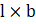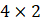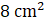Ratio of the sides of the two figures (fig 2:fig 1) = 2:1

Ratio of the areas of the two figures (fig 2:fig 1) = 3:1

Ratio of the perimeter of the two figures (fig 2:fig 1) = 2:1

Question 12.

7 pillars are used to hold a slant iron ladder as shown in the figure. If the distance between every two pillars is 1 m and height of the last piller is 10.5 m. Find the height of pillar.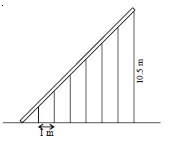Given, Distance between every two pillars = 1 m

Height of the last pillar = 10.5 m

Number of pillars used to hold a slant iron ladder = 7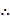Distance between the tip of ladder on floor to the last pillar = 7 × 1 = 7 cm

Height of last pillar = 10.5 cm

Formula Used:

Pythagoras Theorem: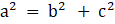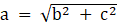Length of slant ladder =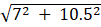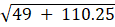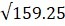= 12.619 cm (ans)

Question 13.

Standing at 5 m apart from a vertical pole of height 3 m, Sudha observed a building at the back of the pillar that tip of the pillar is in line with the top of the building. If the distance between pillar and building is 10 m, estimate the height of the building. [Here height of Sudha is neglected]

Let the height of the building be x cm.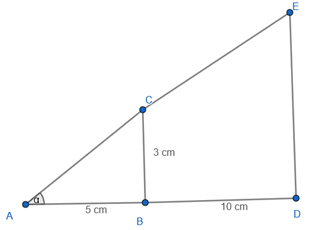In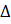ABC,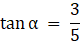InADE,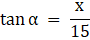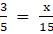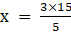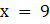Height of building = 9 cm.

Question 14.

Draw a quadrilateral of any measurements. Construct a dilation of scale factor 3. Measure their corresponding sides and verify whether they are similar.

Given, Dilation of scale factor = 3

Let’s draw a square with each side = 3 cm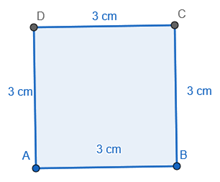Now on dilating the square using scale factor = 3, then

Each side of the square becomes =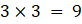cm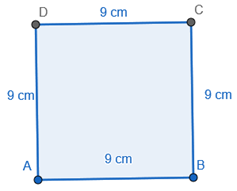We know that two figures are similar if their angles are same.

In the two squares drawn above we know that each angle = 90Hence, both the figures are similar.

###### Exercise 8.2

Question 1.

Cut the bold type English alphabets (capital) and paste in your note book. Draw possible number of lines of symmetry for each of the letter.

(i) How many letters have no linear symmetry?

(ii) How many letters have one line of symmetry?

(iii) How many letters have two lines of symmetry?

(iv) How many letters have more than two lines of symmetry?

(v) Which of them have rotational symmetry?

(vi) Which of them have point symmetry?

(i) 9; F,G,J,L,N,P,Q,R,S

(ii) 13; A,B,C,D,E,K,M,T,U,V,W,Y,Z

(iii)3; H,I,X

(iv) 1;O

(v) 1;O

When a shape it looks the same after some rotation by a partial turn, then its said to have rotational symmetry

(vi) 5; H,J,N,O,X

A figure has point symmetry when it looks the same when up-side-down, or when rotated by 180.

Question 2.

Draw lines of symmetry for the following figures. Identify which of them have point symmetry. Is there any implication between lines of symmetry and point symmetry?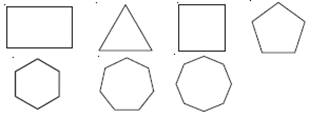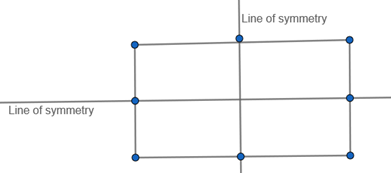Line of symmetry = 2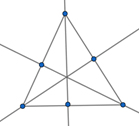Line of symmetry = 3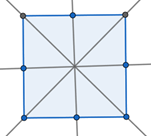Line of symmetry = 8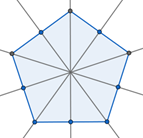Line of symmetry = 10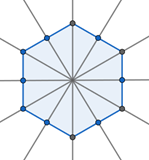Line of symmetry = 12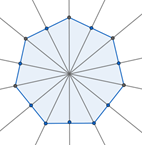Line of symmetry = 14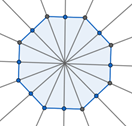Line of symmetry = 16

All of the above figures have point symmetry.

Question 3.

Name some natural objects with faces which have atleast one line of symmetry.

Some natural objects with faces which have atleast one line of symmetry are:

a) Moon

b) Sun

c) Human body

d) Star Fish

e) Plant Leaf

Question 4.

Draw three tessellations and name the basic shapes used on your tessellation.

A tessellation is created when a shape is repeated over and over again to cover the plane without any overlaps or gaps.

Basic figure used-Rectangle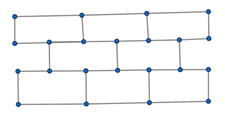Basic figure used-Triangle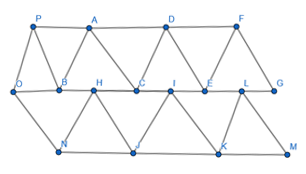Basic figure used-Square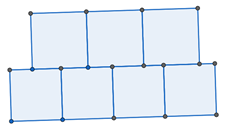## MATHS VIDEOS